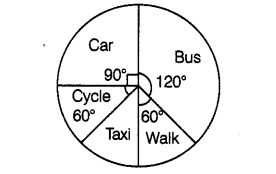# The pie chart given below shows the result

Question:

The pie chart given below shows the result of a survey carried out to find the modes of travel used by the children to go to school. Study the pie chart and answer the questions that follow.(a) What is the most common mode of transport?

(b) What fraction of children travel by car?

(c) If 18 children trfvelby car, how many children took part in the survey?

(d) By which two modes of transport are equal number of children travelling?

Solution:

(a) The central angle is maximum for bus, hence bus is the most common mode of transport.

(b) Fraction of children travelled by car $=\frac{\text { Centralangle }}{360^{\circ}}=\frac{90^{\circ}}{360^{\circ}}$

$=\frac{1}{4}$

(c) We know that, fraction of children travel by car $=\frac{1}{4}$

Hence, total number of children travelled by car $=\frac{1}{4} \times$ Total number of children

$\Rightarrow 18=\frac{1}{4} \times$ Total number of children

Total number of children =18 x 4 = 72

(d) The central angle made up the sectors representing cycle and walk are same. Hence, the cycle and walk are two modes of transport, by which

equal number of children are travelling.